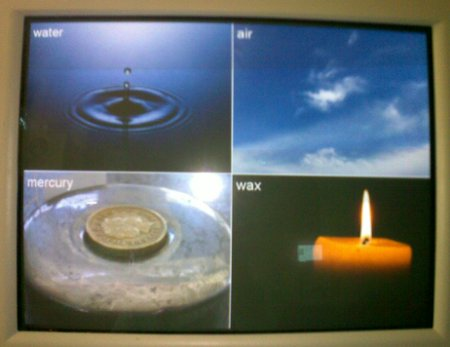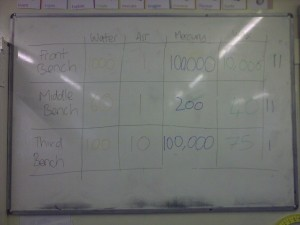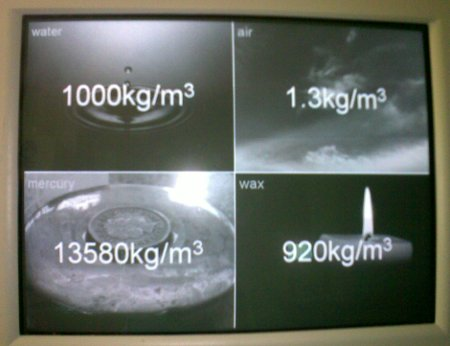# Grains of sand in a breath

I recently saw this tweet:

This immediately didn’t sound right to me, so I decided to investigate.

The volume of the average human breath (the tidal volume) is about half a litre or 0.0005 cubic metres; one half-litre of air is about twenty-two millimoles. One mole contains 6.02×1023 particles, so 22 mmol contains 1.32×1022 particles.

Sand ranges in size, from 0.05 millimetres to one or two millimetres in diameter. Assuming a diameter of 0.5 millimetres, and that sand grains are spherical, gives each grain of sand a volume of 6.54×10?11 cubic metres. Twenty-two millimoles of sand would therefore have a volume of 867 billion cubic metres.

However, this ignores the gaps between the sand particles. The famous mathematician Carl Fredrich Gauss showed that the closest spheres can pack together is with a packing fraction of 74.0%, meaning that 26% of the space is wasted. Our sand would therefore occupy a volume of 1.17 trillion cubic metres rather than the value calculated earlier.

The surface area of the United States (all fifty states, including land and water) is 9.83 trillion square metres. This volume of sand would therefore cover the US to a depth of eleven centimetres, which is nowhere near the height of a eight-storey building. Even if we take “cover the US” to mean only the land and only the forty-eight contiguous states then we still only get to fifteen or sixteen centimetres; and even if we used the largest possible grains of sand we’d still be out by at least two orders of magnitude. The author of the article must be imagining sand grains the size of peas or something.

(Also, the article linked-to in the tweet refers to an average human breath containing 8×1022 particles, which is six times larger than the value I calculated; I think they may have confused inspiratory reserve volume with tidal volume.)

# Estimating density

It turns out that estimating density is something that pupils find really hard.I asked a class of pupils to estimate the density of four substances: air, water, mercury and wax. Before you read any further, try to estimate (in kilograms per metre cubed) the density of these four.In case you can’t read the photograph, their guesses (in kg/m3) were as follows:

• Water – 1000, 60, 100
• Air – 1, 1, 10
• Mercury – 100000, 200, 100000
• Wax – 10000, 40, 75

And the correct values:They were much closer to the correct answer for air than for anything else, but probably only because we’ve discussed it before when looking at mass. Interestingly, taking the geometric mean of the suggested values for mercury gives a value of 12600kg/m3; very close to the correct value of 13580kg/m3, but this is almost certainly a fluke.

So why is estimating density so difficult?

Pupils have far less trouble estimating mass, and less trouble estimating volume (though converting from cm3 to m3 is something they find very taxing). I think the problem comes from a lack of reference points – they’ve all held a kilogram in their hand, and have used metre rulers frequently so can envision a box 1m×1m×1m. The main point of this exercise is to give them reference points. For example: if they calculate the density of a material to be 1200kg/m3 then they know it should sink in water, and something with a density of 1kg/m3 should be lighter than air.